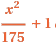# Definite Integral Word Problem

• niravana21

## Homework Statement

A rectangular pool is 10 meters wide and 25 meters long. The depth of water "x" meters from the shallow end of the pool isPart A. Write a left-hand Riemann Sum with 5 terms that approximates the volume of the water in the pool. Is your approximation an underestimate or an overestimate? Explain.

Part B. Write a definite integral that gives the exact volume of the water in the pool.

## The Attempt at a Solution

Don't know where I should even begin. Forgot my book at school and its vacation time:(

Draw a picture looking at the pool from the side. It should be a quadrilateral with base 25 m long, two right angle ends, and end heights $0^2/175+ 1= 1$ and $25^2/175+ 1= 4.57$ meters (not a very deep pool!). A cross section, of width h at distance x from the shallow end, is 10 by 4.57 by h. Take h= 25/n where n is the number of steps you decide to use.